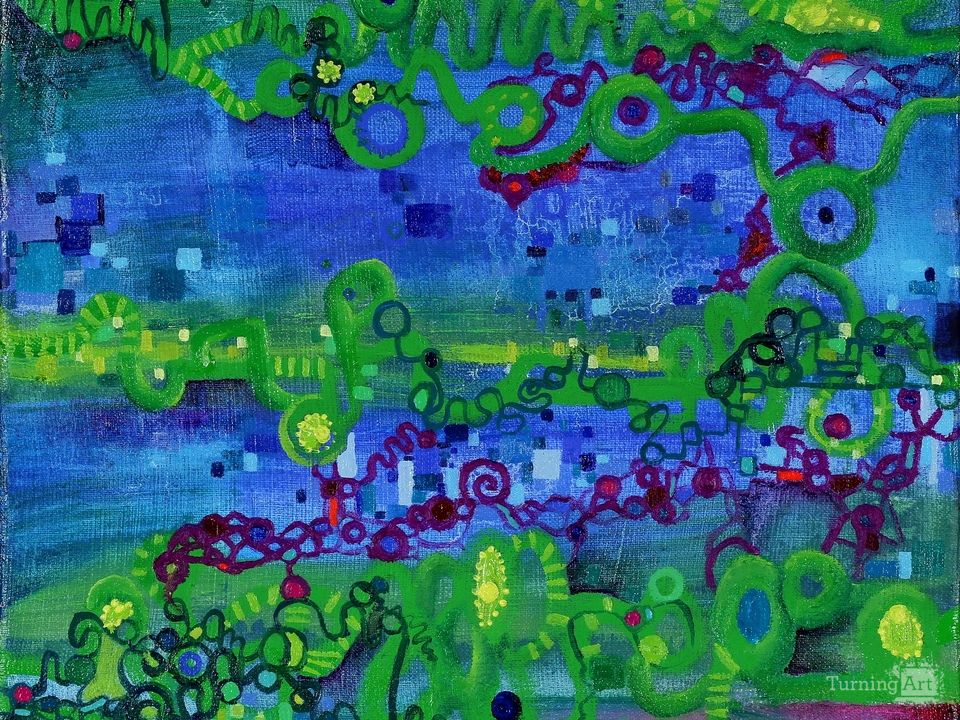# Green Function

Piece description from the artist

A pun on a common path integral. Green functions are a special type of integral that are used in Physics disciplines like the Mathematical physics of Particles and Fields. In calculus, integrals provide methods for adding up functions and trends. Everyday single integrals work in 2 dimensions. A function creates a curve on the plane and solving its integral tells you how much total area the function covers.

Green functions and path integrals can be very useful for examining functions that exist in fields – where instead of an empty paper or box, each piece of the space holding the function has values associated with it (electric field strength, color, temperature, etc). How much of the value does the path pass through as it moves through the field? The Green Function is related to a path integral but is more complex. When statistical possible particle paths are summed up to get an observation, it helps to translate them into topological Feynmann diagrams, work with the algebra of those diagrams and then translate the result back into calculus using the Green Function.

They are generally pretty hard to solve, and as you can see in the painting, the grad students made several failed attempts at numerically cutting up and solving the Green function, and one or two bold souls may have tried to renormalize the surrounding field.

The people interested in Green's Functions are Theoretical physicists and applied mathematicians.

## Other works by Regina Valluzzi